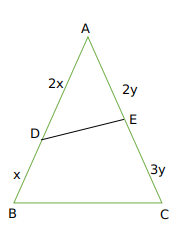Question 64

# Let D and E be points on sides AB and AC, respectively, of a triangle ABC, such that AD : BD = 2 : 1 and AE : CE = 2 : 3. If the area of the triangle ADE is 8 sq cm, then the area of the triangle ABC, in sq cm, is

Solution

We have :Now area of ADE = $$\frac{1}{2}\times\ AD\times\ AE\times\ \sin A$$
=$$\frac{1}{2}\times\ 2x\times\ 2y\times\ \sin A=8$$
we get xy sinA =4
Now Area of triangle ABC = $$\frac{1}{2}AB\times\ AC\times\ \sin A$$
we get $$\frac{1}{2}\times3x\ \times5y\ \sin A=\frac{15}{2}xy\ \sin A=\ \frac{15}{2}\times\ 4$$
we get Area of ABC = 30